# Top Excel Functions For Business

It is no wonder that Excel is still among the most used software in business and finance. Its inexpensiveness, simplicity, and adaptability make it a perfect tool for the job. Not only that but also the fact that you can easily integrate Excel into the Microsoft package makes it very powerful.

What is unsafe about Excel is the fact that when used improperly it can have more drawbacks than advantages. Indeed, Excel allows you to do everything you want. The problem is that in order for you to use all the potentiality of this software you must understand them. For instance, one the greatest advantage and problem of Excel is the fact that it allows you to do things manually.

Therefore, many people pick what seems the easiest path because they don’t know how to automate their spreadsheets. The consequence is that with more manual staff inputted into the spreadsheet more errors will ensue, and the spreadsheet will become a plethora of inaccuracies rather than an effective tool. How to avoid that?

Excel can make or break a career

Since in business and finance the ability to use Excel can make or break a career, in this article I want to show three Excel hacks that any professional should know. Not only because these hacks can be used in multiple ways (your creativity is the limit), but also because these hacks are among the most required into the business industry.

We are going to see how to use three functions: IFERROR, IF statement, and Vlookup. The objective of this article is to show you that also the non-professional can learn how to use more advanced functions quickly and integrate them in the spreadsheets to create automated reports.

In short, we want to avoid manual labor in excel for two main reasons: First, an automated report will be way faster. Second, an automated report will minimize mistakes that usually happen due to manual inputting.

If you want to put into practice these functions, download the Excel sheet here and follow along…

IFERROR AND IF STATEMENT

In this paragraph, we are going to see two main functions: the traditional IF statement and the IFERROR function.

With the IF statements, you can build automated reports. But how does it work? What can we use it for? And what are the limits of this function?

This function allows you to have a result when a condition is met. For instance, if I want to know whether A = B I will have Excel tell me if this is true or false. This is just one of the many possible ways to use this function. But let’s proceed in order; we are going to see how to use the IFERROR function.

IFERROR

This function has an aesthetical and practical purpose, which is to avoid showing into our spreadsheet error messages. Imagine you have to present a report to a client, and the client will use this report in the future.

The spreadsheet is automated and some formulas will show an Error message. How to avoid that?

We use the IFERROR function. For instance, in the case below we have Apple Inc. Income Statement and we want to build a variance report. In short, we want to know what is the difference between 2015 and 2014 financials.

To do that we use a formula, which is: (current year/previous year -1). This formula will give us the percentage of increase or decrease over the previous year like below: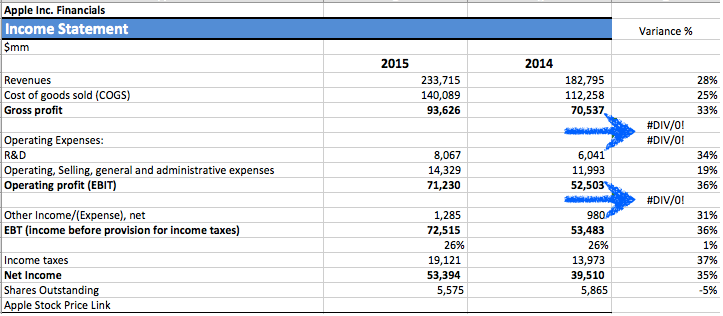As you can see from the picture above, although the formula works, there are certain cells where it shows #DIV/O! We could simply delete these cells, but what if we just make them look empty automatically? In short, we are going to tell excel to show no values in the cells where otherwise would show an error message.

To do so we use the IFERROR formula. This formula will look like the following: IFERROR(value,value_if_error). In the first part of the formula, where it says “value” we are going to input the formula to compute the variance.

In the second part, where it says “value if error” we want to tell excel what to show in the cell in case there is an error. For instance, we want the cell to be empty; therefore we are going to input into the second part of the formula the quotation marks “” which will tell Excel to show the cells as empty ones. See below: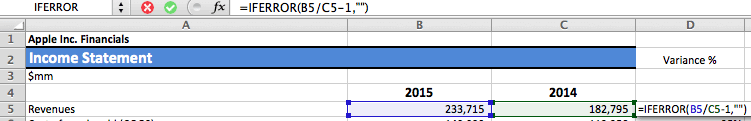You can now drag the formula down by clicking on the bottom right corner of the formula, hold the mouse down and drag the formula up to the desired cell untie you have this formula in the entire column, like below: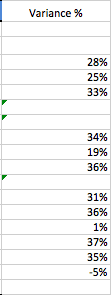As you can see the errors that we saw before are not showing anymore in our report. This is just one of the many ways the IFERROR statement can be used.

IF Statement

Let’s use the IF statement now. Imagine that in the same report we want a formula, which says automatically if 2015 financials increased or decreased compared to 2014. How do we do that?

The IF formula will look like the following: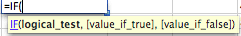Therefore it has three parts: logical test, value if true, and value if false. In the logical test we will decide the condition that will trigger either the value if true or the value if false.

In our case, we want the formula to tell us if the financials for 2015 increased or decreased. Therefore, we are going to input in the logical test that if 2015>2014 (> meaning more than) tell us “increased”, otherwise say, “decreased”. Follow the example below:If you want a word to appear when your condition is met, be sure to open and close the quotation marks “” in fact this will tell Excel to give you back a word. Once you press enter your report will look like the following: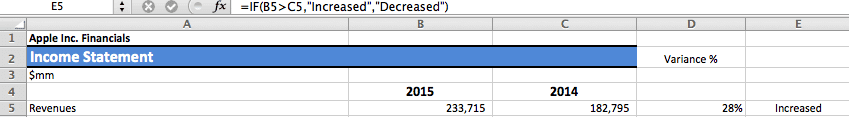As you can see cell E5 automatically shows “increased”. As for the symbol to use into Excel formulas, here the meaning of any of them: > more, < less, = equal.

I showed you just one-way of using the IF statement for simplicity sake, but you can use this function to do many other things as well.

Vlookup Function

This function is among the most used for its simplicity and effectiveness. In fact, this formula is very useful when we have a huge amount of data in our spreadsheet and we want excel to return a certain value within the range of data that we have.

The Vlookup formula will look like the following:Therefore there are four parts in this formula: lookup value, table array, column index and range lookup.

Think of the Vlookup function like a way to produce a search box. Therefore, once you write something in a cell Excel will return something in another cell that you can specify.

In the lookup value we are going input the cell that we want to use as reference. First, we will tell Excel to look for a value next to our reference that will appear in the lookup value. Second, we will select the range of data where we want Excel to look for (table array). Third, we will tell Excel how many columns on the right we want it to move from the reference value found in the range of data. Fourth, And we will tell Excel if we want an exact or approximate match.

For instance, let’s say that we have Apple’s Inc. Income Statement and we want to build a search box that will automatically give us the value we are looking for, see below: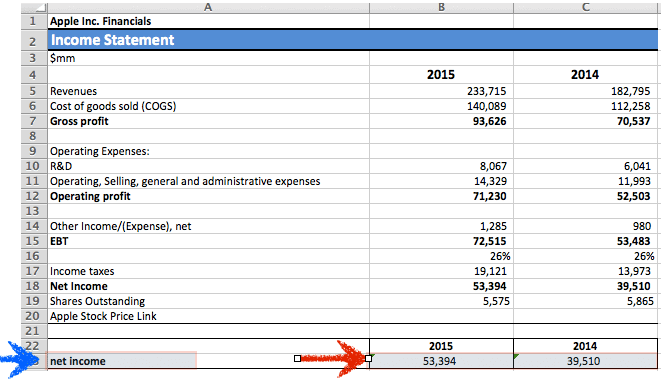In the box where the blue arrow is pointing we are going to write what we are looking for. In the boxes where the red arrow is pointing we want Excel to automatically give us back the values for 2015 and 2014.

How do we write this formula? Follow the example below for 2015: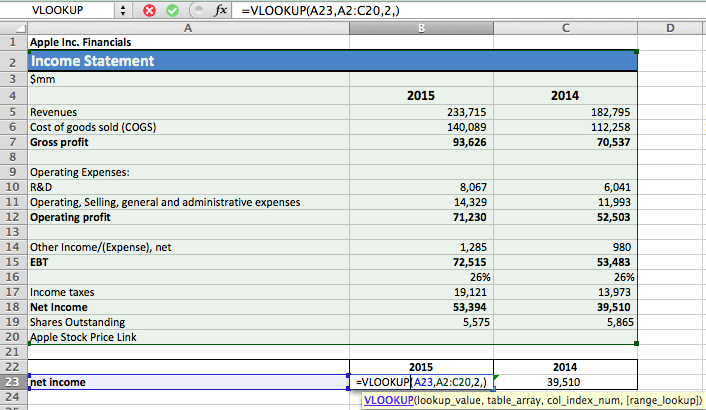The lookup value will be the cell where we are going to input the item we are looking for. In the table array we will select all the data we have on the income statement. And in the column index we will input “2”.

Why do we input that number? Easy, we want Excel to return the value located two columns on the right from the beginning of the selected range of data. As for the range lookup we can leave that blank, but remember to add a coma (follow the example in the picture above).

If we want to create the same formula for 2014 we have to change the column index number to “3”, since we want excel to return us the value for 2014 rather than 2015. Therefore, you have to follow the instructions below: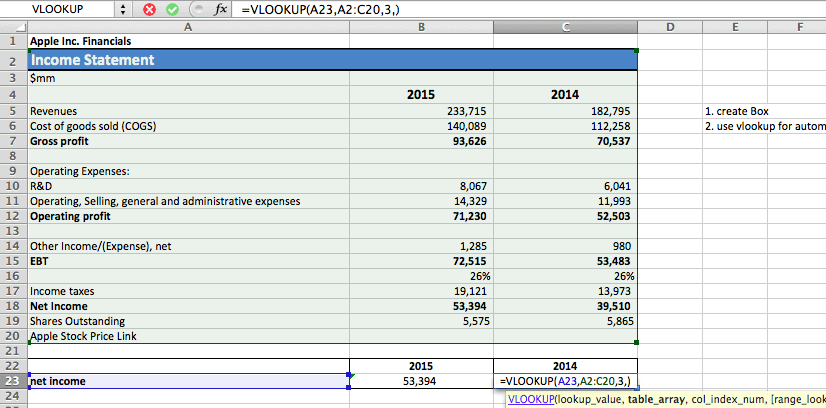As you can see now we have a nice box. This box is automated; therefore it will return the items that we are looking for in 2015 and 2014. For instance, let’s say that we want to know the revenues generated. We go on and write in cell A23 (our lookup value) “revenues” and your report will automatically show you the revenues for 2015 and 2014: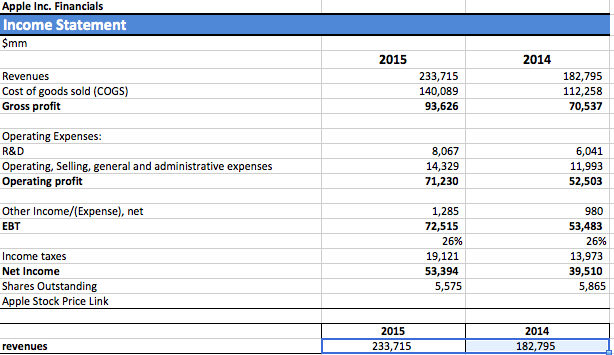Imagine how powerful this function could be if you had a huge set of data. In this article we worked on just some of the hundred of formulas built into Excel.